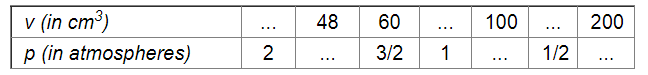# It is known that for a given mass of gas, the volume v varies inversely as the pressure p.`
Question:

It is known that for a given mass of gas, the volume v varies inversely as the pressure p. Fill in the missing entries in the following table:Solution:

Since the volume and pressure for the given mass vary inversely, we have:

$v p=k$

For $v=60$ and $p=\frac{3}{2}$, we have :

$k=60 \times \frac{3}{2}$

$=90$

For $p=2$ and $k=90$, we have :

$2 v=90$

$\Rightarrow v=\frac{90}{2}$

$=45$

For $v=48$ and $k=90$, we have :

$48 p=90$

$\Rightarrow p=\frac{90}{48}$

$=\frac{15}{8}$

For $p=1$ and $k=90$, we have :

$1 v=90$

$\Rightarrow v=\frac{90}{1}$

$=90$

For $v=100$ and $k=90$, we have :

$100 p=90$

$\Rightarrow v=\frac{90}{100}$

$=\frac{9}{10}$

For $p=\frac{1}{2}$ and $k=90$, we have :

$\frac{1}{2} v=90$

$\Rightarrow v=90 \times 2$

$=180$

For $v=200$ and $k=90$, we have :

$200 p=90$

$\Rightarrow p=\frac{90}{200}$

$=\frac{9}{20}$